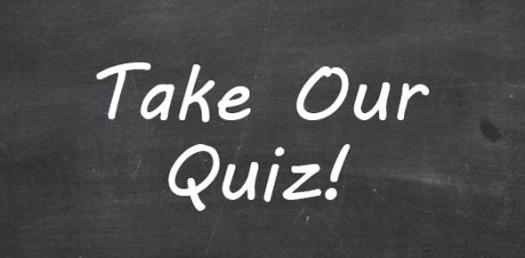# G12 T3 Selfassessment 6.1

Approved & Edited by ProProfs Editorial Team
At ProProfs Quizzes, our dedicated in-house team of experts takes pride in their work. With a sharp eye for detail, they meticulously review each quiz. This ensures that every quiz, taken by over 100 million users, meets our standards of accuracy, clarity, and engagement.
| Written by Frank
F
Frank
Community Contributor
Quizzes Created: 2 | Total Attempts: 754
Questions: 10 | Attempts: 219Settings.

• 1.

### A radioactive nucleus contains 138 neutrons. The nucleus emits an α-particle.How many neutrons are in the nucleus after it has emitted the α-particle?

Explanation
When a radioactive nucleus emits an alpha particle, it loses two protons and two neutrons. Since the question specifically asks for the number of neutrons in the nucleus after the emission, the number of neutrons would decrease by two. Therefore, the correct answer is 136 neutrons.

Rate this question:

• 2.

### An atom of the element lithium has a nucleon number of 7 and a proton number of 3.Which diagram represents a neutral atom of lithium?

• A.

Diagram A

• B.

Diagram B

• C.

Diagram C

• D.

Diagram D

C. Diagram C
Explanation
Diagram C represents a neutral atom of lithium because it shows 3 protons in the nucleus (which corresponds to the proton number of lithium) and 4 neutrons (which can be calculated by subtracting the proton number from the nucleon number). Since the number of protons (positive charge) is equal to the number of electrons (negative charge) in a neutral atom, Diagram C accurately represents a neutral atom of lithium.

Rate this question:

• 3.

### How many protons are there in a neutral atom of

11
Explanation
The number 11 represents the atomic number of an element. In a neutral atom, the number of protons is equal to the atomic number. Therefore, in a neutral atom of element 11, there are 11 protons.

Rate this question:

• 4.

### In the symbol below, A is the nucleon number and Z is the proton number.    What is represented by the symbol?

• A.

An electron

• B.

A neutron

• C.

A nuclide

• D.

An X-ray

C. A nuclide
Explanation
The symbol represents a nuclide. A nuclide is a specific atomic nucleus characterized by its proton number (Z) and nucleon number (A). It is a general term that encompasses all types of atoms, including atoms of different elements and isotopes. The symbol in the question does not indicate the presence of an electron, neutron, or X-ray, but rather represents a nuclide.

Rate this question:

• 5.

### The nucleus of a neutral atom of lithium is represented byHow many protons does the atom contain?

3
Explanation
The nucleus of a neutral atom of lithium is represented by the atomic number, which is equal to the number of protons in the nucleus. In this case, the answer is 3, indicating that the atom of lithium contains 3 protons in its nucleus.

Rate this question:

• 6.

4
• 7.

### The nucleus of a neutral atom of lithium is represented byHow many electrons does the atom contain?

3
Explanation
The nucleus of a neutral atom of lithium is represented by 3. This means that the atom contains 3 electrons. In an atom, the number of protons in the nucleus determines the atomic number, which in turn determines the number of electrons in the atom. Since lithium has an atomic number of 3, it means that it has 3 protons in its nucleus, and therefore 3 electrons orbiting around it.

Rate this question:

• 8.

### How many nucleons are in a nucleus of ?

39
Explanation
The question is asking for the number of nucleons in a nucleus of an unknown element. The answer provided is 39, indicating that there are 39 nucleons in the nucleus.

Rate this question:

• 9.

20
• 10.

### How many electrons are in a nucleus of  if the charge of the atom is +1?Back to top